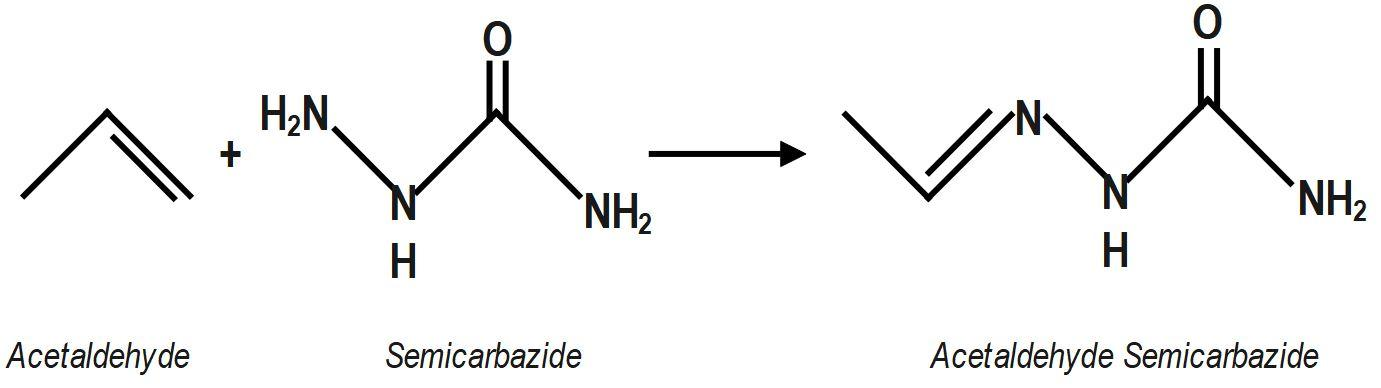Courses
Courses for Kids
Free study material
Offline Centres
MoreLast updated date: 02nd Dec 2023
Total views: 280.5k
Views today: 6.80k

# Acetaldehyde reacts with semicarbazide, product will be:A.$C{{H}_{3}}CH=NNH-CO-N{{H}_{2}}$B.$C{{H}_{3}}CH=NCONHN{{H}_{2}}$C.$C{{H}_{3}}CH=NHN{{H}_{2}}$D.$C{{H}_{3}}-C\left( =O \right)-NH-CON{{H}_{2}}$Verified
280.5k+ views
Hint: We know that Semicarbazone are derivatives of imines and formed by the condensation reaction between an aldehyde or ketone and semicarbazide. Chemical formula of semicarbazide is $N{{H}_{2}}(CONH)N{{H}_{2}}$ the condensation reaction between acetaldehyde $\left( C{{H}_{3}}CHO \right)$ and semicarbazide to get the molecular formula of acetaldehyde semicarbazone.Remember that Semicarbazones are crystalline solids and they are very useful for the identification of aldehydes and ketones by the melting point analysis method. Molecular weight of acetaldehyde semicarbazone is $101\text{ }g/mol.$ Its molecular formula can also be written as: ${{C}_{3}}{{H}_{7}}{{N}_{3}}O.$